Sponsored Link •Weblogs Forum
Generics vs. Dynamic Checking

6 replies on 1 page. Most recent reply: Aug 2, 2005 4:46 PM by Howard Lovatt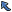Back to Topic List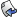Reply to this Topic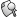Search Forum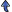Threaded View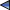Previous Topic Next Topic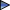Flat View: This topic has 6 replies on 1 page
 Bruce Eckel Posts: 875 Nickname: beckel Registered: Jun, 2003
Generics vs. Dynamic Checking (View in Weblogs)
Posted: Jul 26, 2005 10:18 AMReply
Summary
This entry is a replacement for "When Generics Fail" (which was deleted). In that, I began with casting solution, thinking that there wasn't a generic solution, then Krzysztof Sobolewski corrected me. So instead, I will compare the two solutions.
 AdvertisementBoth solutions work, but with generics you get static type checking -- although you may decide that the syntax is fairly heavy in the generic version.

Here's the old solution, for comparison. It uses Object and casting, instead of generics.

## A test framework

To prevent code duplication and to provide consistency among tests, I've put the basic functionality of the test process into a framework. This is another example of the Template Method design pattern. In this case, however, the core code (that doesn't change) is in the timedTest() method in a separate class Tester, and the Test.test() method is overridden for each particular test:

```//: containers/Test.java
// Framework for performing timed tests on containers.

public abstract class Test {
static int size;
protected static int reps = 50000;
private String name;
// Pass the command-line arguments to this
// method, to modify the default number of reps:
public static void setReps(String[] commandLineArgs) {
if(commandLineArgs.length > 0)
reps = new Integer(commandLineArgs);
}
public Test(String name) { this.name = name; }
public String toString() {
return String.format(Tester.stringField(), name);
}
// Override this Template Method for different tests:
abstract void test(Object container);
} ///:~
```

To use the framework, you create an array of Test objects and an array of container objects to be tested, and pass these to the Tester constructor. When you call run(), each test is run for each one of the container objects in your list, for three different data set sizes (given by the sizes array, which you can also change):

```//: containers/Tester.java
// Applies Test objects to lists of different containers.
import java.util.*;

public class Tester {
public static int fieldWidth = 8;
public int[] sizes = { 10, 100, 1000 };
private Test[] tests;
private String alternateName;
private List containers;
static String stringField() {
return "%-" + fieldWidth + "s";
}
static String doubleField() {
return "%-" + fieldWidth + ".2f";
}
public Tester(Test[] tests, List containers) {
this.tests = tests;
this.containers = containers;
System.out.println(Test.reps + " repetitions");
}
public Tester(Test[] tests, List containers,
String alternateName) {
this(tests, containers);
this.alternateName = alternateName;
}
// Override this to modify pre-test initialization:
protected Object initialize(Object container) {
return container;
}
public void run() {
for(Object container : containers)
timedTest(container);
}
private void timedTest(Object container) {
String name = alternateName;
if(name == null)
name = container.getClass().getSimpleName();
System.out.println("-------------- " + name +
" --------------");

System.out.format(stringField(), "size");
for(Test tst : tests)
System.out.print(tst);
System.out.println();
for(int size : sizes) {
Test.size = size;
System.out.format(stringField(), size);
for(Test tst : tests) {
container = initialize(container);
long start = System.nanoTime();
tst.test(container); // Call the template method
double duration = System.nanoTime() - start;
duration /= (double)Test.size;
System.out.format(doubleField(), duration/1.0e7);
}
System.out.println();
}
}
} ///:~
```

The first constructor just uses the name of the class as the header, but if you want an alternate name, use the second constructor.

If you need to perform special initialization, override the initialize() method. This takes the container object and returns it after it's been initialized. You can see in test() that the result is assigned back to the container reference, and this allows you to replace the container with a completely different initialized container.

Tester also manages the formatting of the output, so it contains a fieldWidth that you can change. This is used to produce format strings.

## Choosing between Lists

All Lists hold their elements in the sequence in which you insert those elements. This program is a performance test for the most essential of the List operations:

```//: containers/ListPerformance.java
// Demonstrates performance differences in Lists.
// {Args: 500} Small to keep build testing short
import java.util.*;
import net.mindview.util.*;

public class ListPerformance {
static Test[] tests = {
void test(Object container) {
List list = (List)container;
for(int i = 0; i < reps; i++) {
list.clear();
for(int j = 0; j < size; j++)
}
}
},
new Test("get") {
void test(Object container) {
List list = (List)container;
for(int i = 0; i < reps; i++) {
for(int j = 0; j < size; j++)
list.get(j);
}
}
},
new Test("set") {
void test(Object container) {
List list = (List)container;
for(int i = 0; i < reps; i++) {
for(int j = 0; j < size; j++)
list.set(j, 1);
}
}
},
new Test("iterate") {
void test(Object container) {
List list = (List)container;
//int passes = reps / 10;
for(int i = 0; i < reps; i++) {
for(Integer t : list)
;
}
}
},
new Test("insert") {
void test(Object container) {
List list = (List)container;
int half = list.size() / 2;
for(int i = 0; i < reps; i++)
}
},
new Test("remove") {
void test(Object container) {
List list = (List)container;
int half = list.size() / 2;
while(list.size() > half)
list.remove(0);
}
},
};
public static void main(String[] args) {
Test.setReps(args);
// Can only do these three tests on an array:
new Tester(new Test[] { tests, tests, tests },
Arrays.asList(1), "Array as List") {
// This will be called before each test. The dummy
// container argument is replaced with an
// non-resizeable array-backed list:
protected Object initialize(Object container) {
Integer[] ia =
new CountingIntegerList(Test.size)
.toArray(new Integer[]{});
return Arrays.asList(ia);
}
}.run();

List> lists = Arrays.asList(
new ArrayList(),
new Vector());
new Tester(tests, lists).run();
}
} /* Output: (Sample)
50000 repetitions
-------------- Array as List --------------
size    get     set     iterate
10      0.12    0.17    0.45
100     0.09    0.12    0.28
1000    0.09    0.12    0.27
50000 repetitions
-------------- ArrayList --------------
size    add     get     set     iterate insert  remove
10      0.31    0.17    0.28    0.36    16.59   12.46
100     0.57    0.16    0.33    0.33    1.67    1.24
1000    0.50    0.15    0.25    0.33    0.22    0.13
size    add     get     set     iterate insert  remove
10      0.67    0.19    0.27    0.38    0.14    0.04
100     0.59    0.41    0.46    0.22    0.05    0.00
1000    0.81    8.11    8.01    0.21    0.02    0.00
-------------- Vector --------------
size    add     get     set     iterate insert  remove
10      0.45    0.14    0.17    0.39    17.12   12.49
100     0.45    0.13    0.19    0.33    1.67    1.25
1000    0.64    0.12    0.16    0.32    0.17    0.13
*///:~
```

Because the container argument is passed as an Object, each Test.test() method must cast the container argument to a Test.

...

## Choosing between Sets

Depending on the behavior you desire, you can choose a TreeSet, a HashSet, or a LinkedHashSet. The following test program gives an indication of the performance trade-off between the implementations:

```//: containers/SetPerformance.java
// {Args: 500}
import java.util.*;

public class SetPerformance {
private static Test[] tests = {
void test(Object container) {
Set set = (Set)container;
for(int i = 0; i < reps; i++) {
set.clear();
for(int j = 0; j < size; j++)
}
}
},
new Test("contains") {
void test(Object container) {
Set set = (Set)container;
for(int i = 0; i < reps; i++)
for(int j = 0; j < size; j++)
set.contains(j);
}
},
new Test("iterate") {
void test(Object container) {
Set set = (Set)container;
for(int i = 0; i < reps * 10; i++) {
Iterator it = set.iterator();
while(it.hasNext())
it.next();
}
}
},
};
public static void main(String[] args) {
Test.setReps(args);
List> sets = Arrays.asList(
new TreeSet(),
new HashSet(),
Tester.fieldWidth = 10;
new Tester(tests, sets).run();
}
} /* Output: (Sample)
50000 repetitions
-------------- TreeSet --------------
10        1.72      0.54      3.73
100       2.49      0.95      2.95
1000      3.73      2.13      3.09
-------------- HashSet --------------
10        0.95      0.46      4.72
100       1.07      0.28      4.19
1000      1.15      0.72      3.90
10        1.32      0.28      5.46
100       1.39      0.29      3.75
1000      1.59      0.62      3.86
*///:~
```

The performance of HashSet is generally superior to TreeSet, but in particular for addition and lookup, the two most important operations. The only reason TreeSet exists is because it maintains its elements in sorted order, so you use it only when you need a sorted Set. Because of the internal structure necessary to support sorting and because iteration is something you're more likely to do, iteration is faster with a TreeSet than a HashSet.

Note that LinkedHashSet is somewhat more expensive for insertions than HashSet; this is because of the extra cost of maintaining the linked list along with the hashed container. However, traversal appears to be more expensive for LinkedHashSet.

## Choosing between Maps

This program gives an indication of the trade-off between Map implementations:

```//: containers/MapPerformance.java
// Demonstrates performance differences in Maps.
// {Args: 500}
import java.util.*;

public class MapPerformance {
private static Test[] tests = {
new Test("put") {
void test(Object container) {
Map map =
(Map)container;
for(int i = 0; i < reps; i++) {
map.clear();
for(int j = 0; j < size; j++)
map.put(j, j);
}
}
},
new Test("get") {
void test(Object container) {
Map map =
(Map)container;
for(int i = 0; i < reps; i++)
for(int j = 0; j < size; j++)
map.get(j);
}
},
new Test("iterate") {
void test(Object container) {
Map map =
(Map)container;
for(int i = 0; i < reps * 10; i++) {
Iterator it = map.entrySet().iterator();
while(it.hasNext())
it.next();
}
}
},
};
public static void main(String[] args) {
Test.setReps(args);
List> maps =
Arrays.asList(
new TreeMap(),
new HashMap(),
new IdentityHashMap(),
new WeakHashMap(),
new Hashtable());
new Tester(tests, maps).run();
}
} /* Output: (Sample)
50000 repetitions
-------------- TreeMap --------------
size    put     get     iterate
10      1.76    0.57    3.64
100     2.51    0.93    3.43
1000    3.83    1.88    3.45
-------------- HashMap --------------
size    put     get     iterate
10      0.88    0.29    4.12
100     0.87    0.26    3.69
1000    1.26    0.56    3.64
size    put     get     iterate
10      1.34    0.38    3.28
100     1.34    0.35    2.34
1000    1.77    0.65    2.25
-------------- IdentityHashMap --------------
size    put     get     iterate
10      1.18    0.68    4.01
100     1.02    0.62    3.43
1000    1.69    1.19    3.12
-------------- WeakHashMap --------------
size    put     get     iterate
10      1.52    0.58    7.47
100     1.39    0.54    6.51
1000    2.00    0.69    1.06
-------------- Hashtable --------------
size    put     get     iterate
10      0.86    0.48    4.89
100     0.78    0.64    3.86
1000    1.14    0.66    3.69
*///:~
```

# The generic solution

Here's the new solution, which uses generics:
```//: containers/Test.java
// Framework for performing timed tests.

public abstract class Test<C> {
static int size;
protected static int reps = 50000;
private String name;
// Pass the command-line arguments to this
// method, to modify the default number of reps:
public static void setReps(String[] commandLineArgs) {
if(commandLineArgs.length > 0)
reps = Integer.parseInt(commandLineArgs);
}
public Test(String name) { this.name = name; }
public String toString() {
return String.format(Tester.stringField(), name);
}
// Override this Template Method for different tests:
abstract void test(C container);
} ///:~

To use the framework, you create an array of Test objects and an array of container objects to be tested, and pass these to the Tester constructor. When you call run(), each test is run for each one of the container objects in your list, for three different data set sizes (given by the sizes array, which you can also change):
//: containers/Tester.java
// Applies Test objects to lists of different containers.
import java.util.*;

public class Tester<C> {
public static int fieldWidth = 8;
public int[] sizes = { 10, 100, 1000 };
private List<Test<C>> tests;
private List<C> containers;
private String alternateName;
static String stringField() {
return "%-" + fieldWidth + "s";
}
static String doubleField() {
return "%-" + fieldWidth + ".2f";
}
public Tester(List<Test<C>> tests, List<C> containers) {
this.tests = tests;
this.containers = containers;
System.out.println(Test.reps + " repetitions");
}
public Tester(List<Test<C>> tests, List<C> containers,
String alternateName) {
this(tests, containers);
this.alternateName = alternateName;
}
// Override this to modify pre-test initialization:
protected C initialize(C container) {
return container;
}
public void run() {
for(C container : containers)
timedTest(container);
}
private void timedTest(C container) {
String name = alternateName;
if(name == null)
name = container.getClass().getSimpleName();
System.out.println("-------------- " + name +
" --------------");
System.out.format(stringField(), "size");
for(Test tst : tests)
System.out.print(tst);
System.out.println();
for(int size : sizes) {
Test.size = size;
System.out.format(stringField(), Test.size);
for(Test<C> tst : tests) {
container = initialize(container);
long start = System.nanoTime();
// Call the template method:
tst.test(container);
double duration = System.nanoTime() - start;
duration /= Test.size; // Normalize
System.out.format(doubleField(), duration / 1.0e7);
}
System.out.println();
}
}
} ///:~

The first constructor just uses the name of the class as the header, but if you want an alternate name, use the second constructor.
If you need to perform special initialization, override the initialize() method. This takes the container object and returns it after its been initialized. You can see in test() that the result is assigned back to the container reference, and this allows you to replace the container with a completely different initialized container.
Tester also manages the formatting of the output, so it contains a fieldWidth that you can change. This is used to produce format strings.
Choosing between Lists
All Lists hold their elements in the sequence in which you insert those elements. This program is a performance test for the most essential of the List operations:
//: containers/ListPerformance.java
// Demonstrates performance differences in Lists.
// {Args: 500} Small to keep build testing short
import java.util.*;
import net.mindview.util.*;

public class ListPerformance {
static List<Test<List<Integer>>> tests = Arrays.asList(
void test(List<Integer> list) {
for(int i = 0; i < reps; i++) {
list.clear();
for(int j = 0; j < size; j++)
}
}
},
new Test<List<Integer>>("get") {
void test(List<Integer> list) {
for(int i = 0; i < reps; i++) {
for(int j = 0; j < size; j++)
list.get(j);
}
}
},
new Test<List<Integer>>("set") {
void test(List<Integer> list) {
for(int i = 0; i < reps; i++) {
for(int j = 0; j < size; j++)
list.set(j, 1);
}
}
},
new Test<List<Integer>>("iterate") {
void test(List<Integer> list) {
for(int i = 0; i < reps; i++) {
Iterator<Integer> it = list.iterator();
while(it.hasNext())
it.next();
}
}
},
new Test<List<Integer>>("insert") {
void test(List<Integer> list) {
int half = list.size() / 2;
for(int i = 0; i < reps; i++)
}
},
new Test<List<Integer>>("remove") {
void test(List<Integer> list) {
int half = list.size() / 2;
while(list.size() > half)
list.remove(0);
}
}
);
public static void main(String[] args) {
Test.setReps(args);
List<List<Integer>> dummy =
new ArrayList<List<Integer>>(1);
// Can only do these three tests on an array:
new Tester<List<Integer>>(tests.subList(1, 4), dummy,
"Array as List") {
// This will be called before each test. The
// dummy container argument is replaced with
// a non-resizeable array-backed list:
@Override protected List<Integer> initialize(
List<Integer> container) {
Integer[] ia = Generated.array(Integer.class,
new CountingGenerator.Integer(), Test.size);
return Arrays.asList(ia);
}
}.run();
List<List<Integer>> lists =
Arrays.<List<Integer>>asList(
new ArrayList<Integer>(),
new Vector<Integer>());
new Tester<List<Integer>>(tests, lists).run();
}
} /* Output: (Sample)
50000 repetitions
-------------- Array as List --------------
size    get     set     iterate
10      0.12    0.18    0.41
100     0.09    0.13    0.25
1000    0.09    0.14    0.25
50000 repetitions
-------------- ArrayList --------------
size    add     get     set     iterate insert  remove
10      0.39    0.17    0.24    0.31    18.05   13.55
100     0.57    0.16    0.32    0.28    1.91    1.46
1000    0.72    0.17    0.31    0.35    0.19    0.15
size    add     get     set     iterate insert  remove
10      0.80    0.35    0.35    0.37    0.16    0.05
100     0.87    0.57    0.72    0.24    0.06    0.00
1000    0.93    8.16    13.05   0.20    0.03    0.00
-------------- Vector --------------
size    add     get     set     iterate insert  remove
10      0.48    0.15    0.27    0.40    18.36   13.43
100     0.44    0.13    0.26    0.34    1.78    1.36
1000    0.58    0.13    0.25    0.37    0.21    0.16
*///:~

To compare array access to container access (primarily against ArrayList), a special test is created for arrays by wrapping an array as a List using Arrays.asList( ). Only three of the tests can be performed in this case, because you cannot insert or remove elements from an array. Notice that the initialize() method throws away the container argument (so that argument is a dummy object) and creates a completely new object using Generated.array() (which was defined in the Arrays chapter).
In main(), the definition of lists requires an explicit type argument specification to give a hint to the Arrays.asList() method call.
Choosing between Sets
Depending on the behavior you desire, you can choose a TreeSet, a HashSet, or a LinkedHashSet. The following test program gives an indication of the performance trade-off between the implementations:
//: containers/SetPerformance.java
// {Args: 500}
import java.util.*;

public class SetPerformance {
static List<Test<Set<Integer>>> tests = Arrays.asList(
void test(Set<Integer> set) {
for(int i = 0; i < reps; i++) {
set.clear();
for(int j = 0; j < size; j++)
}
}
},
new Test<Set<Integer>>("contains") {
void test(Set<Integer> set) {
for(int i = 0; i < reps; i++)
for(int j = 0; j < size; j++)
set.contains(j);
}
},
new Test<Set<Integer>>("iterate") {
void test(Set<Integer> set) {
for(int i = 0; i < reps * 10; i++) {
Iterator<Integer> it = set.iterator();
while(it.hasNext())
it.next();
}
}
}
);
public static void main(String[] args) {
Test.setReps(args);
List<Set<Integer>> sets = Arrays.<Set<Integer>>asList(
new TreeSet<Integer>(),
new HashSet<Integer>(),
Tester.fieldWidth = 10;
new Tester<Set<Integer>>(tests, sets).run();
}
} /* Output: (Sample)
50000 repetitions
-------------- TreeSet --------------
10        2.12      0.67      5.01
100       2.97      0.97      3.32
1000      4.60      2.48      3.40
-------------- HashSet --------------
10        1.01      0.32      4.36
100       0.98      0.30      3.89
1000      1.25      0.88      4.20
10        1.59      0.43      4.73
100       1.60      0.33      3.33
1000      1.83      0.68      3.05
*///:~

Choosing between Maps
This program gives an indication of the trade-off between Map implementations:
//: containers/MapPerformance.java
// Demonstrates performance differences in Maps.
// {Args: 500}
import java.util.*;

public class MapPerformance {
static List<Test<Map<Integer,Integer>>> tests =
Arrays.asList(
new Test<Map<Integer,Integer>>("put") {
void test(Map<Integer,Integer> map) {
for(int i = 0; i < reps; i++) {
map.clear();
for(int j = 0; j < size; j++)
map.put(j, j);
}
}
},
new Test<Map<Integer,Integer>>("get") {
void test(Map<Integer,Integer> map) {
for(int i = 0; i < reps; i++)
for(int j = 0; j < size; j++)
map.get(j);
}
},
new Test<Map<Integer,Integer>>("iterate") {
void test(Map<Integer,Integer> map) {
for(int i = 0; i < (reps * 10); i++) {
Iterator it = map.entrySet().iterator();
while(it.hasNext())
it.next();
}
}
}
);
public static void main(String[] args) {
Test.setReps(args);
List<Map<Integer,Integer>> maps = Arrays.asList(
new TreeMap<Integer,Integer>(),
new HashMap<Integer,Integer>(),
new IdentityHashMap<Integer,Integer>(),
new WeakHashMap<Integer,Integer>(),
new Hashtable<Integer,Integer>());
new Tester<Map<Integer,Integer>>(tests, maps).run();
}
} /* Output: (Sample)
50000 repetitions
-------------- TreeMap --------------
size    put     get     iterate
10      2.13    0.71    5.18
100     3.11    0.95    3.16
1000    4.34    1.94    3.43
-------------- HashMap --------------
size    put     get     iterate
10      0.95    0.37    4.51
100     0.91    0.28    3.94
1000    1.40    0.62    4.01
size    put     get     iterate
10      1.37    0.47    3.62
100     1.32    0.38    2.45
1000    1.98    0.75    2.50
-------------- IdentityHashMap --------------
size    put     get     iterate
10      1.22    0.69    4.23
100     1.02    0.63    3.87
1000    2.04    1.34    3.41
-------------- WeakHashMap --------------
size    put     get     iterate
10      1.60    0.59    7.45
100     1.49    0.54    6.81
1000    2.26    0.76    1.07
-------------- Hashtable --------------
size    put     get     iterate
10      1.08    0.51    5.45
100     0.99    0.67    4.08
1000    1.50    0.82    3.81
*///:~

```

 Edoardo Comar Posts: 7 Nickname: edoardo Registered: Apr, 2003
Re: Generics vs. Dynamic Checking Posted: Jul 27, 2005 1:03 AMReply
I didn't like the way the original article has been deleted.
I found this blog entry by chance after I started looking for the original.
You should ahve posted the content as follow-up in the orginal thread which had much more exposure, IMO.

 Isaac Gouy Posts: 527 Nickname: igouy Registered: Jul, 2003
Re: Generics vs. Dynamic Checking Posted: Jul 27, 2005 10:47 AMReply
Both solutions work, but with generics you get static type checking -- although you may decide that the syntax is fairly heavy in the generic version

1) Actually it's one of those tiny examples that make you wonder if the only reason we've created a class is that Java hasn't provided a useful alternative.

Given the option, instead of Test class, maybe we'd choose to represent the tests as an array of test name - method tuples, something like:
`void main(String[] args){   let n = TestRepetitions(args);   let mapTests = [         ("put",          (Map<int,int> map, int size) => {            for(int i = 0; i < n; i++) {               map.clear();               for(int j = 0; j < size; j++)                  map.put(j, j);            }         }      )                ,("get",          (Map<int,int> map, int size) => {            for(int i = 0; i < n; i++)               for(int j = 0; j < size; j++)                  map.get(j);         }      )             ,("iterate",          (Map<int,int> map, int size) => {            for(int i = 0; i < (n * 10); i++) {               let it = map.entrySet().iterator();               while(it.hasNext())                  it.next();            }         }      )          ];`

2) And (unless we had some serious resource constraints) wouldn't we separate calculation from presentation?

The Tester method timedTest has a fixed test presentation order because we must populate the container object before doing the other tests - but we might reasonably expect that the tests should be presented get,put,iterate (not put,get,iterate).

Wouldn't it be cleaner to accumulate test results and bother about how to display them later?
`var int[] containerSizes = [10, 100, 1_000];<C> List<(String,String,int,double)> ContainerTestResults(      C[] containers,       (String, (C,int)->void)[] tests){                  let results = new ArrayList();                            for(C container : containers){               for(int size : containerSizes){                              for (int i=0; i<tests.size; i++){                                        (String testName, (C,int)->void test) = tests[i];            let start = System.nanoTime();            test(container,size);                        double duration = System.nanoTime() - start;                                      results.add( (containerName, testName, size, duration/size) );         }      }   }   return results;}// now do something with the list of result tuplesContainerTestResults(   containers: [new TreeMap(), new HashMap(), new LinkedHashMap(),                new IdentityHashMap(), new WeakHashMap(), new Hashtable()],   tests: mapTests    );`

 Bruce Eckel Posts: 875 Nickname: beckel Registered: Jun, 2003
Re: Generics vs. Dynamic Checking Posted: Jul 27, 2005 11:34 AMReply
> Given the option, instead of Test class, maybe we'd choose
> to represent the tests as an array of test name -
> method tuples, something like:
`> void main(String[] args){>    let n = TestRepetitions(args);What language are you using here? Are these compiling and running examples?> 2) And (unless we had some serious resource constraints)> wouldn't we separate calculation from presentation?> > Wouldn't it be cleaner to accumulate test results and> bother about how to display them later? This is a very interesting observation; I was struggling with some other problems which I think relate to this same issue. I'll have to sleep on this and see what happens.`

 Isaac Gouy Posts: 527 Nickname: igouy Registered: Jul, 2003
Re: Generics vs. Dynamic Checking Posted: Jul 27, 2005 12:00 PMReply
What language are you using here? Are these compiling and running examples?

Nice - http://nice.sourceforge.net/
They were, unless I changed something in the post form.

 Isaac Gouy Posts: 527 Nickname: igouy Registered: Jul, 2003
Re: Generics vs. Dynamic Checking Posted: Jul 28, 2005 1:35 PMReply
`//Here's the definition for package testervar int[] containerSizes = [10, 100, 1_000];int TestRepetitions(String[] args) =   args.size > 0 ? Integer.parseInt(args) : 50_000;<C> List<(String,String,int,double)> ContainerTestResults(      C[] containers,       (String,(C,int)->void)[] tests){                  let results = new ArrayList();                            for(C container : containers){      let containerName = notNull(container).getClass.getName;                  for(int size : containerSizes){                              for (int i=0; i<tests.size; i++){                                        (String testName,(C,int)->void test) = tests[i];            let start = System.nanoTime();            test(container,size);                        double duration = System.nanoTime() - start;                                      results.add( (containerName,testName,size,duration/size) );         }      }   }   return results;}// (same as before) the text for package tester.mapsimport tester;void main(String[] args){   let n = TestRepetitions(args);      let mapTests = [         ("put",          (Map<int,int> map, int size) => {            for(int i = 0; i < n; i++) {               map.clear();               for(int j = 0; j < size; j++)                  map.put(j, j);            }         }      )                ,("get",          (Map<int,int> map, int size) => {            for(int i = 0; i < n; i++)               for(int j = 0; j < size; j++)                  map.get(j);         }      )             ,("iterate",          (Map<int,int> map, int size) => {            for(int i = 0; i < (n * 10); i++) {               let it = map.entrySet().iterator();               while(it.hasNext())                  it.next();            }         }      )              ];   // now do something with the list of result tuples   ContainerTestResults(         containers: [new TreeMap(), new HashMap(), new LinkedHashMap(),                   new IdentityHashMap(), new WeakHashMap(), new Hashtable()],      tests: mapTests       );} `

 Howard Lovatt Posts: 321 Nickname: hlovatt Registered: Mar, 2003
Re: Generics vs. Dynamic Checking Posted: Aug 2, 2005 4:46 PMReply
Isaac Gouy has suggested a good improvement to the test framework and coded it in Nice. However his suggestions code just as well (if not better) in Java:
```package bruceeckelstester;

import java.util.*;

interface Test {
void test( Map< Integer, Integer > map, int size );
}

public enum Main implements Test {
PUT {
public void test( final Map< Integer, Integer > map, final int size ) {
for ( int i = 0; i < n; i++ ) {
map.clear();
for ( int j = 0; j < size; j++ )
map.put( j, j );
}
}
},
GET {
public void test( final Map< Integer, Integer > map, final int size ) {
for ( int i = 0; i < n; i++ )
for ( int j = 0; j < size; j++ )
map.get( j );
}
},
ITERATE {
public void test( final Map< Integer, Integer > map, final int size ) {
for ( int i = 0; i < (n * 10); i++ )
for ( final Integer value : map.values() )
;
}
};

private static int n;

public static void main( final String[] args ) {
n = testRepetitions( args );
System.out.println(
containerTestResults(
new TreeMap< Integer, Integer>(),
new HashMap< Integer, Integer>(),
new IdentityHashMap< Integer, Integer>(),
new WeakHashMap< Integer, Integer>(),
new Hashtable< Integer, Integer>() ) );
}

private static int testRepetitions( final String[] args ) {
return args.length > 0 ? Integer.parseInt( args[ 0 ] ) : 10;
}

private static final int[] containerSizes = { 10, 100, 1000 };

private static class Result {
String containerName;
String testName;
int size;
double duration;
public String toString() {
return "Container = " + containerName + ", Test = " + testName + ", Size = " + size + ", Rate = " + (duration / size)  + " ns/element\n";
}
}

private static List< Result > containerTestResults( final Map< Integer, Integer >... containers ) {
final List< Result > results = new ArrayList< Result >();
for ( final Map< Integer, Integer > container : containers )
for ( final int size : containerSizes )
for ( final Test test : values() ) {
final Result result = new Result();
result.containerName = container.getClass().getName();
result.testName = test.toString();
result.size = size;
final double start = System.nanoTime();
test.test( container, size );
result.duration = System.nanoTime() - start;
}
return results;
}
}
```

 Flat View: This topic has 6 replies on 1 pagePrevious Topic Next TopicWeb Artima.com